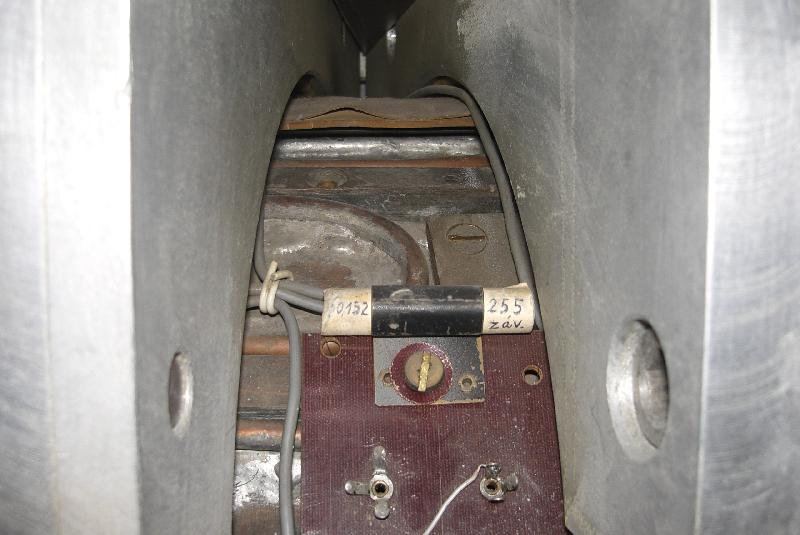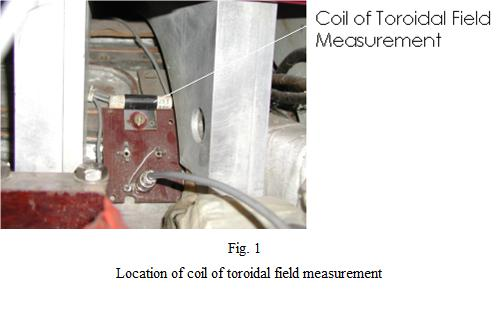# Coil of BT MeasurementCoil of B(t) measurement

## Principle of Operation

The principle of magnetic field measurements by magnetic coil is explained in section Mirnov coils.

## Characterization and Parameters of Sensor

As it is evident from its name, this coil is used for measurements of toroidal magnetic field and is part of basic tokamak diagnostics, being implemented into DAS1 data acquisition system. The coil itself is made of 255 turns in total, each having diameter of 10 mm. Coil is located on the low field side of the tokamak, as can be seen from fig. 1. This sensor can be used for local magnetic field measurements. Geometrical properties of this coil determine its effective area to be equal to 0.02 m2. However, poloidal coordinate (and thus its exact radius from major axis) of this coil is hard to determine and thus it is not possible to state any relation between B magnitude measured by this coil to B magnitude in the center of the tokamak. It was thus necessary to calibrate this coil with other sensor of toroidal magnetic field.

In tokamak GOLEM, there is a large loop encircling chamber of tokamak in poloidal direction (radius of 0.145 m). Even thought it is not currently part of tokamak diagnostic system, it can be used for measurements of average BT. It was measured that sensitivity of small coil of toroidal field measurement is 5.98 – 6.06 times lower than the sensitivity of the large loop, if the measurements of B averaged through the whole poloidal cross-section are considered. This implies that effective area of the small coil is 6 times smaller than the area of the loop and determines the effective area of the coil to be equal to 0.011 m2, as far as averaged B measurements are considered.The small coil placed on the low field side of the tokamak can thus be used either for measurement of local BT (by assuming effective area to be 0.02 m2) or for measurement of averaged BT (by assuming effective area to be 0.011 m2).